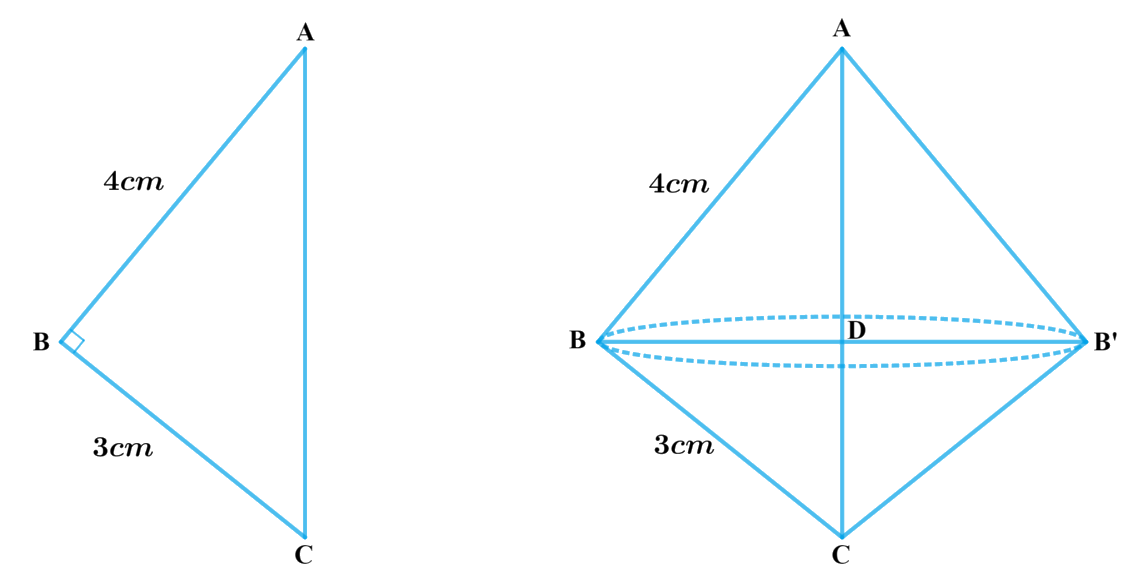In the verge of coronavirus pandemic, we are providing FREE access to our entire Online Curriculum to ensure Learning Doesn't STOP!

# Ex.13.5 Q2 Surface Areas and Volumes Solution - NCERT Maths Class 10

Go back to  'Ex.13.5'

## Question

A right triangle, whose sides are $$3 \,\rm{cm}$$ and $$4\,\rm{cm}$$ (other than hypotenuse) is made to revolve about its hypotenuse. Find the volume and surface area of the double cone so formed. (Choose value of π as found appropriate.)

Video Solution
Surface Areas And Volumes
Ex 13.5 | Question 2

## Text Solution

What is Known?

A right triangle whose sides are $$3 \,\rm{cm}$$ and $$4\,\rm{cm}$$ (other than hypotenuse) is made to revolve about its hypotenuse to form cone.

What is Unknown?

The volume and surface area of the double cone formed.

Reasoning:

Draw a figure to visualize the double cone formedIn order to find the volume and surface area, we need to find $$BD$$ or radius of the double cone

From the figure it’s clear that $$BD \bot AC$$

To find $$BD$$

(i) We first find $$AC$$ using Pythagoras theorem

\begin{align}A{C^2} &= A{B^2} + B{C^2}\\AC &= \sqrt {A{B^2} + B{C^2}} \end{align}

(ii) Using AA criterion of similarity

Prove

\begin{align}&{\Delta ABC}\sim{\Delta BDC}\\&{ \frac{{AB}}{{BD}} = \frac{{AC}}{{BC}}}\end{align}

(Corresponding Sides of similar triangles are in proportion)

\begin{align}\text{Radius or}\, BD = \frac{{AB}}{{BC}} \times BC\end{align}

Since we know $$AB, AC$$ and $$BC; BD$$ can be found out

Since double cone is made by joining $$2$$ cones by their bases

Therefore Volume of double cone $$=$$ Volume of Cone $$ABB$$ $$+$$ Volume of Cone $$BCB$$

We will find the volume of the cone by using formulae;

Volume of the cone\begin{align} = \frac{1}{3}\pi {r^2}h\end{align}

where $$r$$ and $$h$$ are the radius and height of the cone respectively.

Visually from the figure it’s clear that CSA of double cone includes CSA of both the cones

Therefore, CSA of double Cone $$=$$ CSA of cone $$ABB’ +$$ CSA of cone $$BCB’$$

We will find the CSA of the cone by using formulae;

CSA of frustum of a cone$$= \pi rl$$

where $$r$$ and $$l$$ are the radius and slant height of the cone respectively.

Steps:

In $$\Delta ABC$$ right-angled at $$B$$

\begin{align}A{C^2} &= A{B^2} + B{C^2}\\AC &= \sqrt {{{\left( {3cm} \right)}^2} + {{\left( {4cm} \right)}^2}} \\&= \sqrt {9c{m^2} + 16c{m^2}} \\&= \sqrt {25c{m^2}} \\&= 5cm\end{align}

Consider $$\Delta ABC$$ and $$\Delta BDC$$

\begin{align}\angle ABC &= \angle CDB = 90^\circ {\rm{ }}\left( {BD \bot AC} \right)\\\angle BCA &= \angle BCD{\rm{}}\left( {{\rm{common}}} \right)\end{align}

By AA criterion of similarity $$\Delta ABC \sim \Delta BDC$$

Therefore,

\begin{align}\frac{{AB}}{{BD}} = \frac{{AC}}{{BC}}\end{align} (Corresponding sides of similar triangles are in proportion)

\begin{align}BD &= \frac{{AB \times BC}}{{AC}}\\&= \frac{{3cm \times 4cm}}{{5cm}}\\&= \frac{{12}}{5}cm\\&= 2.4cm\end{align}

Volume of double cone $$=$$ Volume of Cone ABB’ $$+$$ Volume of Cone BCB’

\begin{align}&= \frac{1}{3}\! \times \!\pi {(BD)^2} \times AD + \frac{1}{3}\pi {(BD)^2}\! \times\! DC\\&= \frac{1}{3} \times \pi {(BD)^2}\left[ {AD + DC} \right]\\&= \frac{1}{3} \times \pi {(BD)^2} \times AD\\&= \frac{1}{3} \times 3.14 \times 2.4cm \times 2.4cm \times 5cm\\&= \frac{{90.432}}{3}c{m^3}\\&= 30.144c{m^3}\\&= 30.14c{m^3}\end{align}

CSA of double Cone $$=$$ CSA of cone ABB’ $$+$$ CSA of cone BCB’

\begin{align}&= \pi\!\times\!BD \!\times\!AB\!+\!\pi\! \times\!BD\!\times\!BC\\&= \pi \times BD\left[ {AB + BC} \right]\\&= 3.14 \times 2.4cm \times \left[ {3cm + 4cm} \right]\\&= 3.14 \times 2.4cm \times 7cm\\&= 52.752c{m^2}\\&= 52.75c{m^2}\end{align}

Learn from the best math teachers and top your exams

• Live one on one classroom and doubt clearing
• Practice worksheets in and after class for conceptual clarity
• Personalized curriculum to keep up with school